Setting Up Curve Sets

This section provides an overview of operation codes and discusses how to:

• Define the curve sets data sources.

• Define the curve sets market issues.

• Define the curve sets derived data.

• Define the curve sets generic data.

To set up curve sets, set up the Data Source page. On this page, you specify the data type that you use. This data type field determines which of the following additional pages you set up:

• Generic Data page.

• Market Issues page.

• Derived Data page.

Pages Used to Set Up Curve Sets

Page Name

Definition Name

Usage

Data Source

YC_DATA_SRC_PNL

select Financial Services Industries, then select Interest Rate Environment, then select Dataset Definition, then select Curve Sets, then select Data Source

Set up the data source within the system.

Generic Data

YC_GENERIC_PNL

select Financial Services Industries, then select Interest Rate Environment, then select Dataset Definition, then select Curve Sets, then select Generic Data

Use when creating term structures from generic data sets.

Market Issues

YC_MRKTYIELD_PNL

select Financial Services Industries, then select Interest Rate Environment, then select Dataset Definition, then select Curve Sets, then select Market Issues

Used to create a synthetic curve composed of numerous market issues with different attributes as and assign those combined market issues to a new data source.

Derived Data

YC_COMPST_PNL

select Financial Services Industries, then select Interest Rate Environment, then select Dataset Definition, then select Curve Sets, then select Derived Data

Used to create composite curves.

Curve Sets - Notes

YC_DATA_NOTES_PNL

select Financial Services Industries, then select Interest Rate Environment, then select Dataset Definition, then select Curve Sets, then select Notes

Use page for setup notes.

Understanding Operation Codes

Operation codes are used to calculate new data based on existing data sets. These codes are used on the Derived Data page.

Image: Operation codes

This chart illustrates some examples of operation codes: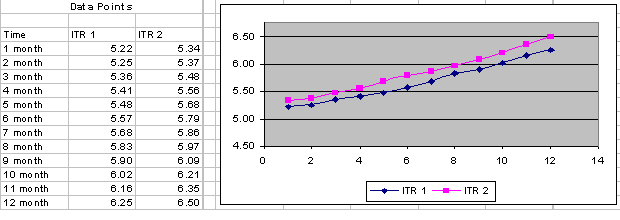The operation codes are:

• Average (AVG)

• Also

• Difference (DIFF)

• Minimum (MIN)

• Maximum (MAX)

• Shift

• Shift Range

• Splice

• Sum

AVG

Image: AVG

Average returns the average value between two values. For example, following is a plot of internal transfer rate 1 and internal transfer rate 2, with the average of the two data sets making up the third plot as the average of the two data sets: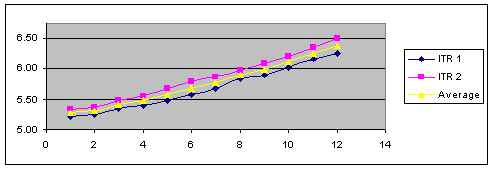ALSO

Also adds a new source to an already calculated operation and repeats this calculation with the result of the previously calculated result and the new source. Also is most likely used in conjunction with the AVG operation code to average an additional data source with the previously averaged values.

For example, the ITR 1 curve and the ITR 2 curve are averaged by using the AVG operation code. In addition, you want to calculate the average between this newly calculated average (comprised of the averaged data set ITR 1 and ITR 2) and the Corp BB curves. This is done by using the ALSO operation code.

`(ITR 1 + ITR 2) /2 = Average value `
`(Average + Corp BB) /2 = Also value `

Image: ALSO

The following graph illustrates the ALSO operation code: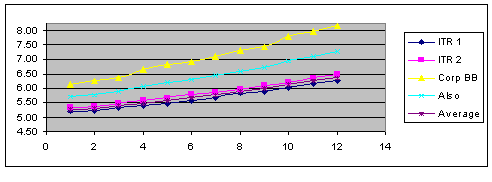Note: Average represents the average between ITR 1 and ITR 2.Also represents the average between average and Corp BB.

DIFF

Image: DIFF

This operation code calculates the absolute difference between two yields. DIFF subtracts the lower from the higher value and displays the new value. No negative values can result from this operation. The following graph illustrates the DIFF operation code.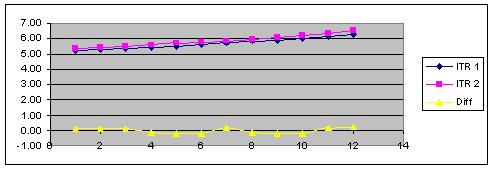MIN

Image: MIN

MIN calculates the absolute minimum value from two sources. This operation code compares two data sets and selects the lowest representative data point between the two data sets. The following graph illustrates the MIN operation code.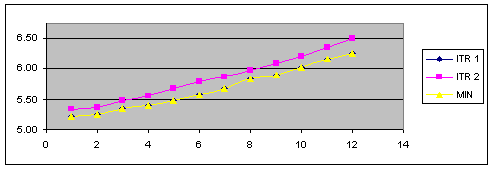MAX

Image: MAX

MAX calculates the absolute maximum value from two sources. This operation code compares two data sets and selects the greatest representative data point between the two data sets. The following graph illustrates the MAX operation code.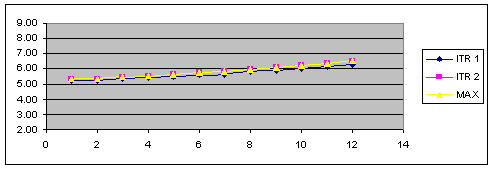SHIFT

Image: SHIFT

The SHIFT operation code allows the user to apply a basis point shift to an existing data set. The basis point shift is applied to the entire data set. The following graph illustrates the SHIFT operation code.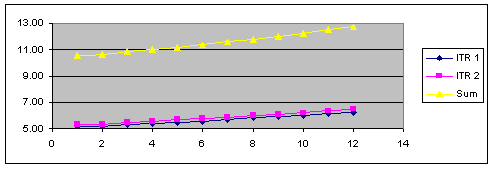SHIFT RANGE

Image: SHIFT RANGE

SHIFT RANGE allows the user to apply a basis point shift to an existing data set that is within a specified time frame. The time frame is specified with a start maturity and an end maturity, which are defined in days, months, or years rather than as actual dates. The following graph illustrates the SHIFT RANGE operation code.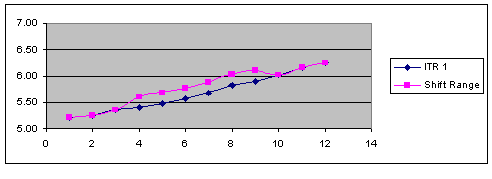SPLICE

Splice divides an existing curve into a new curve with two or more data sources or types of interpolants.

Note: A curve's interpolant can be spliced only if Global Interpolant isnot selected.

Image: SPLICE

The following graph illustrates the SPLICE operation code.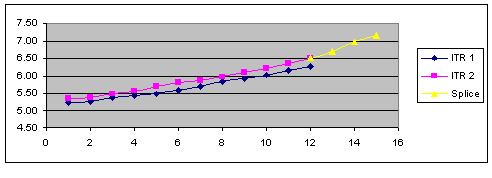SUM

Image: SUM

The SUM operation code adds the data points of two data sets and creates a third data set with the totals. The following graph illustrates the SUM operation code.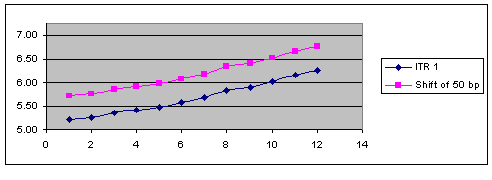Data Source Page

Use the Data Source page (YC_DATA_SRC_PNL) to set up the data source within the system.

Image: Data Source page

This example illustrates the fields and controls on the Data Source page. You can find definitions for the fields and controls later on this page.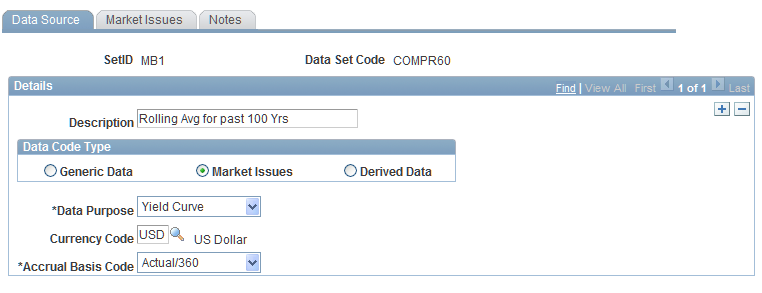Specify the type of data sets from which you construct the yield curves. Options are:

• Generic Data.

The source data is composed of user-defined data. You supply the x-y axis coordinates and units of measure for this data.

• Market Issues (default).

The market rate instrument data is assigned to this source data.

• Derived Data.

Create your own data from more than one market source. Your choice determines the type of tab that follows the Data Source tab.

Specify the purpose for these data set points. Options are Yield Curve, Commodity, Credit Spread, Foreign Exchange, Volatility, orOther. Specify the currency code and the accrual basis code. Set up the second tab (subsequent Curve Sets page). This tab varies according to the selection in theData Type field.

Data Source Page for Rolling Averages

Use Data Source page for rolling averages

Image: Data Source page for rolling averages

This example illustrates the fields and controls on the Data Source page for rolling averages. You can find definitions for the fields and controls later on this page.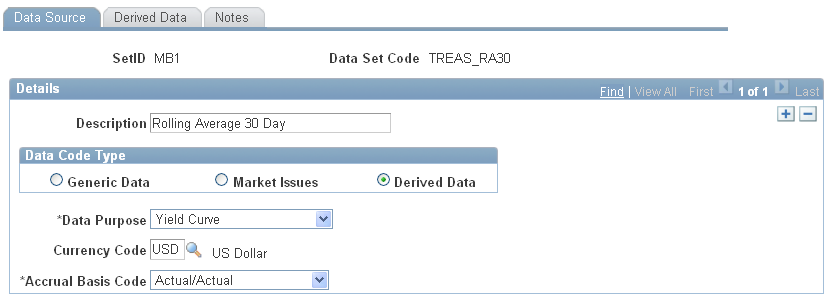Market Issues Page

Image: Market Issues page

This example illustrates the fields and controls on the Market Issues page. You can find definitions for the fields and controls later on this page.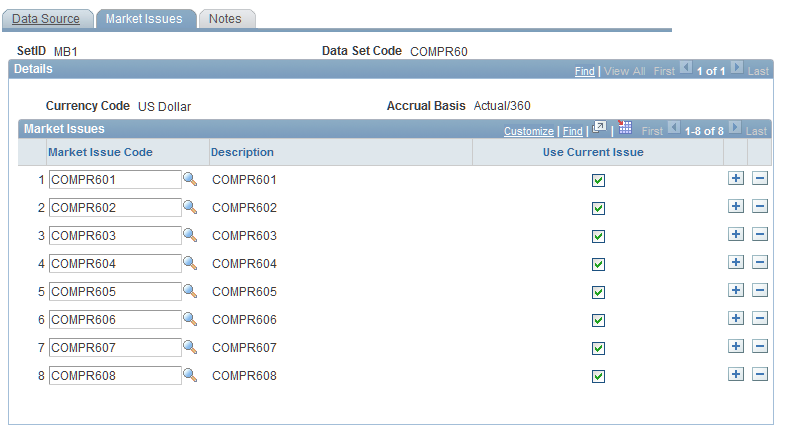Select a market issue code where you are prompted. Select from codes that you previously set up on the Market Issues page. Specifying a code in this field enables you to create a synthetic curve comprised of numerous market issues that have different attributes and assign those combined market issues to a new data source.

There is a minimum number of data points (market issues that you must select for the curve set definition) to generate unique curve results. This minimum number varies depending on the yield curve interpolation method: 1 for step, 2 for linear, 3 for hermite cubic, and 5 for cubic spline.

Derived Data Page

Use the Derived Data page (YC_COMPST_PNL) to used to create composite curves.

Image: Derived Data page

This example illustrates the fields and controls on the Derived Data page. You can find definitions for the fields and controls later on this page.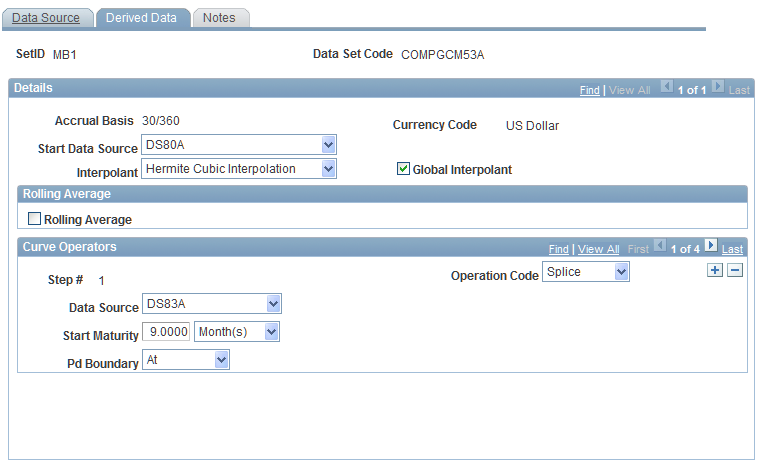To set up the derived data page, specify the source data that you want to use for this composite curve in the Start Data Source field. Should the construction of data points that you specify require more source data, select an interpolant to use in fitting a curve to the data points. Select theGlobal Interpolant check box to interpolate all the data in the new set in the same manner as the source data that you specify in theStart Data Source field.

If you want to calculate rolling averages based on historic interest rate information, select the Rolling Average check box. This activates two other fields, theLag Frequency andRolling Average Frequency fields that enable you to select dates from previous yield curves.

Next, set up the curve operators. The Step # field indicates the source data that is affected by the chosen operation and helps you keep track of the operations that are performed and the segments of the composite curve that are impacted). Select an operation code to determine operation on the current (and possibly previous) data set that is given in the data source prompt box. Options are:Average, Also, Difference, Max Min, Shift, Shift-Range, Splice, andSum. Depending on the selection, the following fields may appear:

Derived Data Page

Use the Derived Data page

Image: Derived Data page

This example illustrates the fields and controls on the Derived Data page. You can find definitions for the fields and controls later on this page.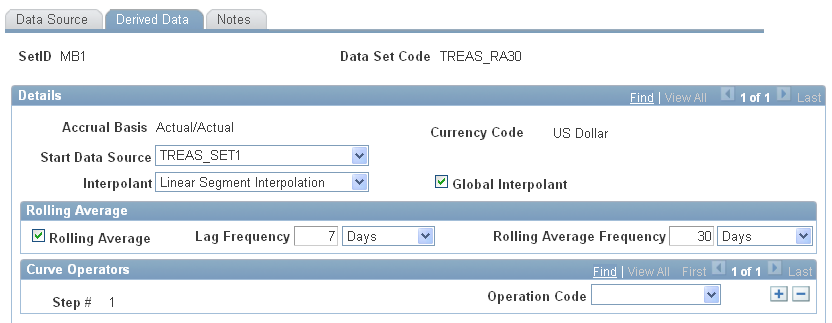Generic Data Page

Use the Generic Data page (YC_GENERIC_PNL) to use when creating term structures from generic data sets.

Image: Generic Data page

This example illustrates the fields and controls on the Generic Data page. You can find definitions for the fields and controls later on this page.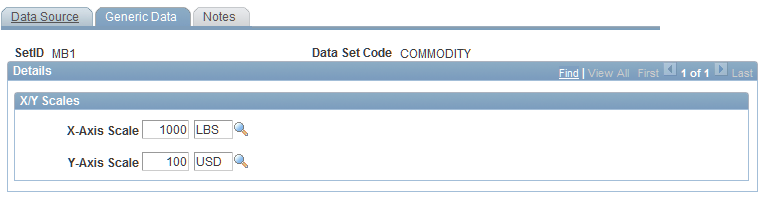Enter values for the x and y axis. These values are previously set up in PeopleSoft EPM by using the Unit of Measure page. Create generic data sets to provide maximum flexibility for curve generation of desired user defined parameters and data. You provide the data inputs and measures that are needed to define the data set.